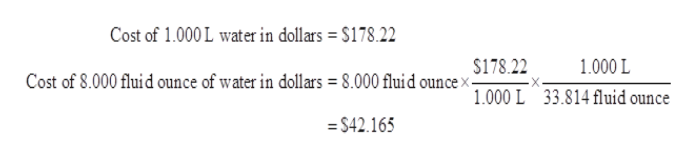# if a 1.000 L bottle of water cost 134 Euros and the exchange rate is 1 Euro= \$1.33, what is the cost in dollars for 8.000 fluid ounces of the bottle of water?

Question
26 views

if a 1.000 L bottle of water cost 134 Euros and the exchange rate is 1 Euro= \$1.33, what is the cost in dollars for 8.000 fluid ounces of the bottle of water?

check_circle

Step 1

Unit conversion  is a means converting between the units of same quantity. Different units of a particular quantity are related through conversion factors. For example, 1 L = 1000 mL. '

In this, both mL and L are the units for volume and 1000 is the conversion factor.

Step 2

As per question,

Cost of 1.000 L bottle of water = 134 Euros

To convert it into dollars, the given exchange rate is 1 Euro = \$ 1.33

Cost of 1.000 L of bottle in dollars can be calculated as below:

Step 3

Now, the cost in dollars is required for a bottle  containing 8.000 fluid ounce of water while the cost calculated is for 1.000 L of water.

Both L and fluid ounce are the units of volume.

The conve...help_outlineImage TranscriptioncloseCost of 1.000 L water in dollars \$178.22 \$178.22 1.000 L Cost of 8.000 fluid ounce of water in dollars = 8.000 fluid ounce> 1.000 L 33.814 fluid ounce = S42.165 fullscreen

### Want to see the full answer?

See Solution

#### Want to see this answer and more?

Solutions are written by subject experts who are available 24/7. Questions are typically answered within 1 hour.*

See Solution
*Response times may vary by subject and question.
Tagged in

### Chemistry